#INFOSWMM

# QA/QC Process for the Import of an XP-SWMM model to InfoWorks ICM 5.5 or ICM 6

The following section shows how the XP-SWMM file (MyXP.XP) was converted to an ICM 6 model using the ICM SWMM 6 import tools.

The XP-SWMM model was converted to ICM 6 using a combination of the import process in ICM of a SWMM5 input file (MySWMM5File.INP ) and an Extensive QA/QC process using the .XP file of XP-SWMM (MyXP.XP). Figure 1 shows how the file MySWMM5File.INP looks inside of SWMM 5.1. Figure 2 shows the model summary of the file MySWMM5File.INP which was exported in SWMM 5 format from XP-SWMM. Figure 3 shows the Import process in ICM for any SWMM5 file. Figure 4 show how the file MySWMM5File.INP looks like ICM after the Import. Figure 5 shows the element summary in ICM of the imported file MyXP.XP. The number of links, nodes, Subcatchments and diversions match the input file and the summary shown in Figure 2. There are approximately 7000 nodes, links, diversions and Subcatchments in the model.

After import into ICM the imported data was checked using the Network Validation tool (Figure 6). If there were any import errors a log file would have been created. However, all data imported without errors and the log file was blank for ICM 6 import. ICM has many more node and link parameters than in XP-SWMM so there are some validation errors. The validation errors are shown in Figure 7 and are related to the node of ICM needing chamber, ground and flood levels. You can click on the warning message as shown in Figure 8 to bring up the node grid so that the few validation errors can be fixed. As a further check the .XP file was used to check any ICM Validation Messages as shown in Figure 9.

The import tool in ICM does not import the Rainfall or DWF patterns from the SWMM5 file. The hyetographs and DWF patterns have to be separately copied and pasted from the SWMM5 file to the Rainfall Event CSV file and Waste Water Patter CSV file in ICM. Figure 10 shows the DWF Patterns used in the file MySWMM5File.INP . Figure 11 shows the cumulative Rainfall Hyetographs used in the file MySWMM5File.INP . The Rainfall hyetograph have to be converted to rainfall intensity and multiplied by the storm event total as defined in the file MyXP.XP  The rainfall totals for the 2, 5, 10, 25, 50 and 100 year storm events are shown in Figure 12. Figure’s 13 and 14 show the DWF Pattern and ICM Rainfall Event corresponding to the values shown in Figures 10 and 11, respectively. The connection between the Pattern and Rainfall CSV files and the imported network in ICM is illustrated in Figure 15.

The XP-SWMM model in SWMM 5 format has many irregular natural channels modeled as prismatic HEC-RAS transects. Figure 16 shows an example transect view. The imported network in ICM has these transects as channels with a shape named after the transect (Figure 17). The channels can be either used as channel in ICM or converted to a River Reach with 2D connection using the ICM Channel/River Reach conversion tool (Figure 18).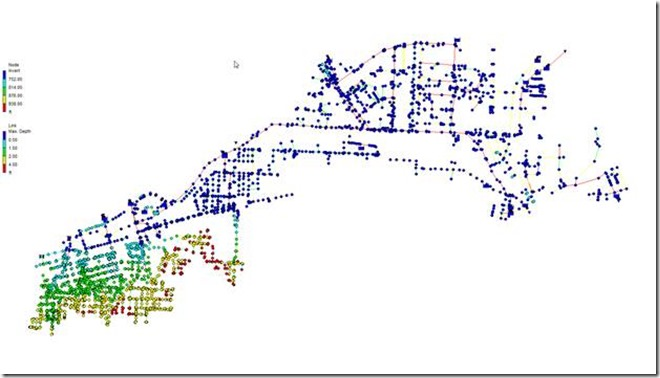Figure 1. How the MySWMM5File.INP looks inside of SWMM5

The three types of runoff surfaces in the XP-SWMM model are imported with the physical data on the Subcatchment Tab. Examples of the physical data are the slope, width, Subcatchment area and percent imperviousness for each Subcatchment (Figure 19). The Impervious surface with depression storage is imported as a Fixed Runoff Volume Type with a non-zero initial loss and the SWMM Routing Model. The Impervious surface without depression storage is imported as a Fixed Runoff Volume Type with a zero initial loss and the SWMM Routing Model. The pervious surface with depression storage is imported as a Green-Ampt Runoff Volume Type with a non-zero initial loss and the SWMM Routing Model. The three types of runoff surface can be seen on the Runoff Tab of the Subcatchment Grid.Figure 2. MySWMM5File.INP File Summary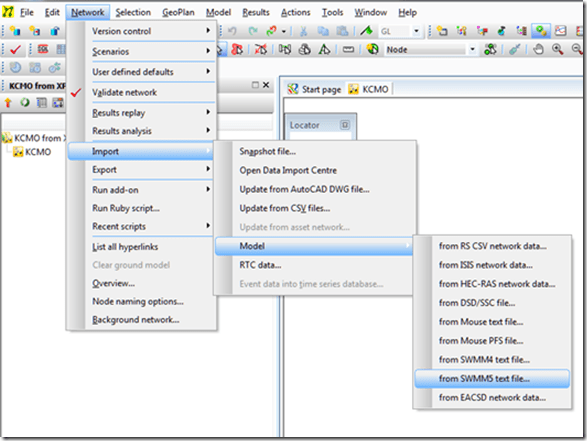Figure 3. The tools use to import the file MySWMM5File.INP to ICM.Figure 4. How the file MySWMM5File.INP looks like ICM after Import.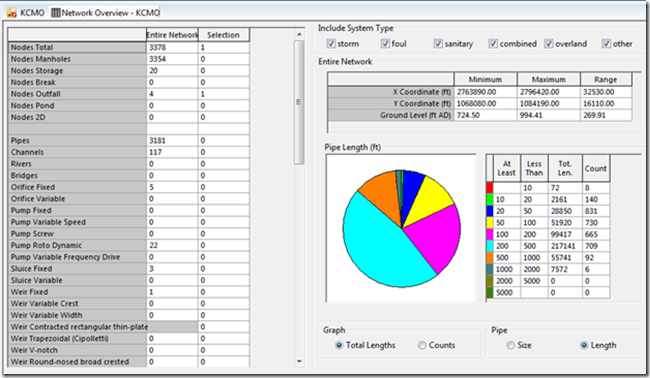Figure 5. Summary of the file MySWMM5File.INP after import into ICM.Figure 6. The Network Validation Tool checks the imported file for data errors.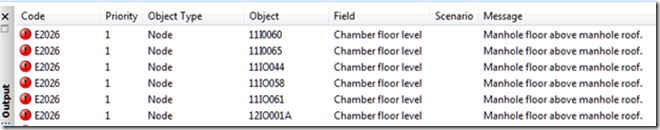Figure 7. Import Data Errors are shown in Output.Figure 8. Click on Warning Message and the location is shown on the screen.Figure 9. The XP file was used to check any ICM Validation Messages.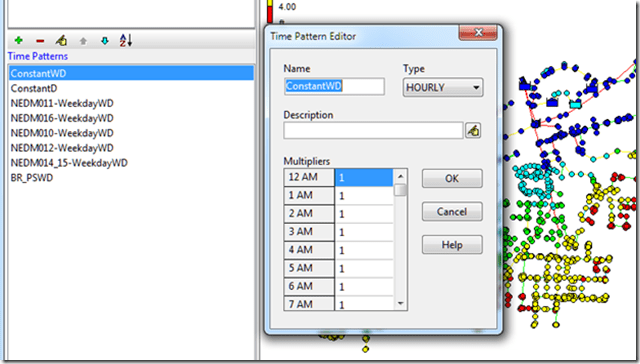Figure 10. The DWF Patterns from XP-SWMM as they look in SWMM5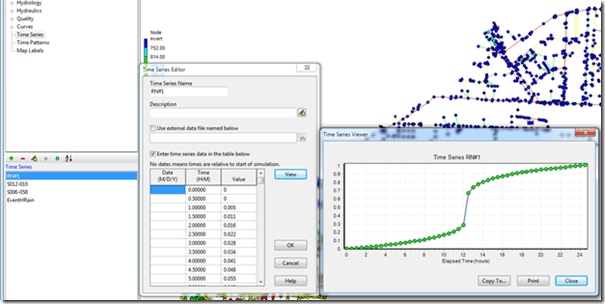Figure 11. The Rainfall Hyetograph in XP-SWMM.Figure 12. The 2, 5, 10, 25, 50 and 100 year Storm Totals used In XP-SWMM.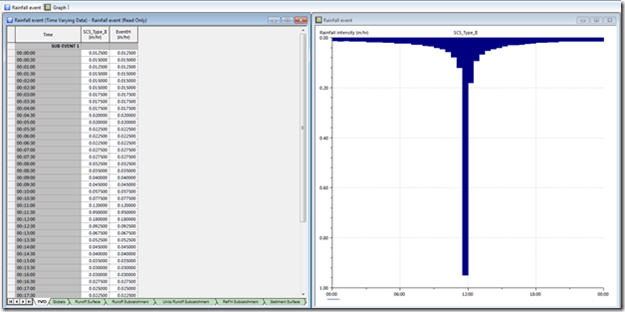Figure 14. The Converted Hyetograph as it looks in the ICM Rainfall Event.Figure 15. Imported XP-SWMM Network, Rainfall and DWF Patterns in ICM.Figure 16. Prismatic Channel Transects in XP-SWMM.Figure 17. Imported Transects from XP-SWMM are Channel Shapes in ICM.Figure 18. You can convert the Channels to River Reaches with Banks and 2D connections using the Channel to River Reach Tool.Figure 19. The XP-SWMM Hydrology is imported as Fixed or Green-Ampt for Impervious and Pervious Areas.

Categories: #INFOSWMM, ICM, InfoSWMM